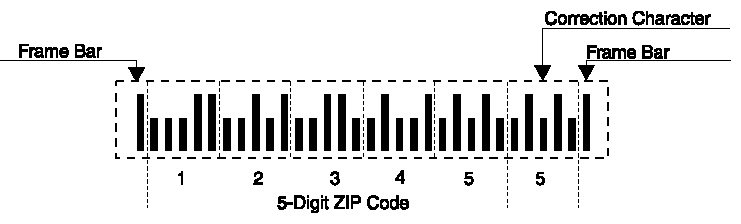## Postnet Specification

Whether it represents five-, nine-, or eleven-digit ZIP Code information, the POSTNET barcode is always printed in a format that begins and ends with a frame bar (full or tall bar). To ensure POSTNET accuracy during mail processing, a correction character (five bars) must be included immediately before the rightmost frame bar of all POSTNET barcodes. The correction character is always the digit that, when added to the sum of the other digits in the barcode, results in a total that is a multiple of 10. For example, the sum of the ZIP+4 barcode 12345-6789 is 45. Adding a correction character of 5 results in the sum of the 10 digits being a multiple of 10.### Code Elements

The basic elements of the POSTNET barcode are binary digits, represented as full bars and half bars (or tall bars and short bars). A full bar represents “1” (one) and a half bar represents “0” (zero).

` `

### Numeric Binary Code Value Value

```    1          00011
2          00101
3          00110
4          01001
5          01010
6          01100
7          10001
8          10010
9          10100
0          11000
```

### Code Characters

Each code character is made up of five bars, which together represent a single numeric digit. Specific combinations of two full bars and three half bars represent the digits 0 through 9. Only the 10 combinations shown in the table are valid code characters - they represent all possible combinations of two full bars and three half bars.
These combinations are central to the error-recovery of POSTNET because the system interprets as an error the combination of five bars containing other than two full and three half bars.

### Bar Position Weights

Except for zero, the numeric value of each valid combination of five bars can be determined by adding the “weights” of the two positions occupied by the full bars (“1s”). From left to right, the bar positions are weighted 7, 4, 2, 1, and 0.

For example, the combination 01010 contains a full bar in the second position (weight 4) and in the fourth position (weight 1). Adding 4 and 1 yields 5 - the assigned value of this combination. The only exception is the combination 11000, which has a total weight of 11 but is assigned a value of zero.

### Bar Spacing (Pitch)

Horizontal Spacing

The nominal horizontal spacing (pitch), defined as a bar and a space, must be limited to 22 bars (±2 bars) per inch when measured over any ½-inch (0.500- inch) portion of the barcode. The horizontal spacing at 24 bars per inch is 0.0416 inch and 0.050 inch at 20 bars per inch. There should be a clear space of at least 0.012 inch, but not more than 0.040 inch, between individual bars

The dimensions described below should be maintained for five-, nine-, and eleven-digit POSTNET barcodes so that our barcode sorters can accommodate the tolerances encountered with different printing technologies.

### Bar dimensions

Tall bars should be between 0.115 and 0.135 inches tall. Short bars should between 0.040 and 0.060 inches tall. Bar widths should be between 0.015 and 0.025 inches wide.

### Five-Digit ZIP Code (32 Bars - A Field)

The distance from the leading edge of the first (leftmost) bar to the leading edge of the thirty-second (rightmost) bar should be at least 1.245 inches. The distance from the leading edge of the first bar to the trailing edge of the thirty-second bar should not exceed 1.625 inches.

### Nine-Digit ZIP+4 Code (52 Bars - C Field)

The distance from the leading edge of the first (leftmost) bar to the leading edge of the fifty-second (rightmost) bar should be at least 2.075 inches. The distance from the leading edge of the first bar to the trailing edge of the fifty-second bar should not exceed 2.625 inches.

### Eleven-Digit Delivery Point Code (62 Bars - C Prime Field)

The distance from the leading edge of the first (leftmost) bar to the leading edge of the sixty-second (rightmost) bar should be at least 2.495 inches. The distance from the leading edge of the first bar to the trailing edge of the sixty-second bar should not exceed 3.125 inches.